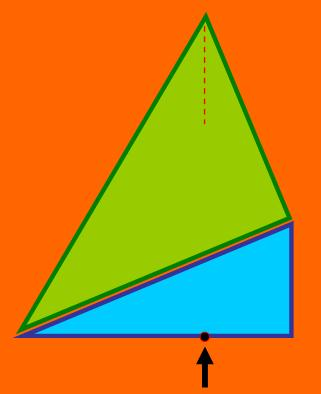Two right-angled triangles are joined as shown in the diagram.

The green triangle has side lengths of 65, 52 and 39 cm and the blue triangle has side lengths of 52, 48 and 20 cm.

An object is dropped vertically from the top of the green triangle hitting the base by the arrow.

Where does it hit the base of the blue triangle?

In what proportion is the blue base split and how far has the object fallen?

This problem does not require a calculator or any special formula.